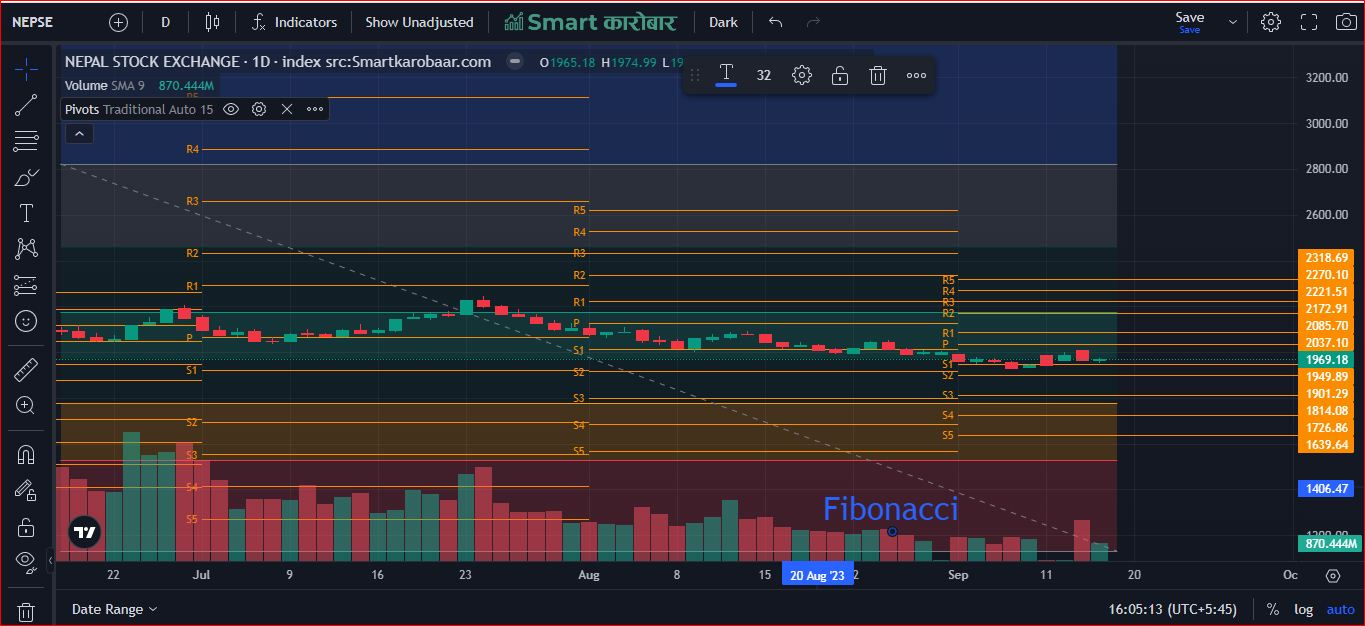What is Fibonacci in share market?

In the context of the stock market & financial market Fibonacci is the technical mathematical analysis tools and principles based on the Fibonacci sequence and ratio. The Fibonacci sequences are a Mathematical in which each number is the sum of the two preceding once   starting with 0 and 1. Fibonacci numbers, occasionally referred to as retracements by traders, are popular key parts of technical analysis in trading. Fibonacci number sequence is well-known among traders who rely on technical analysis since it is based on the critical numbers established by a mathematician named Leonardo Pisano, who was given the nickname 'Fibonacci' in the 13th century. The actual numbers are not as important as the mathematical relationships expressed in terms of ratios. In trading, when equities move up or down sharply, they tend to retrace their steps before making further moves. These are known as retracements and can be anticipated using mathematical ratios. The Fibonacci number series, in which one number can be calculated by adding the two before it. As a result, the series begins with 0 and continues with 2, 3, 5, 8, 13, 21, and so on. If you choose a number from the series and divide it by the number immediately before it, you will always receive an approximate ratio of 1.618. Identifying Support and Resistance: Fibonacci retracement levels on price charts give potential support and resistance levels. Traders utilize these levels to forecast where a stock's price will reverse or consolidate, allowing them to make more informed judgments on entry and exit points.Fibonacci retracements and extensions are two often utilized Fibonacci methods in stock market analysis:

Fibonacci Retracements: These are used to identify possible price trend levels of support and resistance. Traders and investors use Fibonacci retracement levels to predict where the price of a stock will reverse or consolidate. The most common retracement levels are 38.2%, 50%, and 61.8%, while additional levels such as 23.6% and 78.6% are sometimes utilized. Because they are based on ratios drawn from the Fibonacci sequence, traders feel that these levels signify potential reversal points.

Fibonacci Extensions: When a stock is in an uptrend, these are utilized to determine prospective price goals. Traders frequently utilize Fibonacci extension levels to determine profit goals when a stock is rising. The most typical extension levels, which are likewise derived from the Fibonacci sequence, are 127.2%, 161.8%, and 261.8%.Significance of Fibonacci in Smart Karobaar App

• Identifying Support and Resistance:   The smart karobaar App provide Identifying support to Resistance. Fibonacci retracement levels on price charts give potential support and resistance levels. Traders utilize these levels to forecast where a stock's price will reverse or consolidate, allowing them to make sensible decisions on entry and exit points.
• Price Target projection: In an upswing, Fibonacci extension levels assist traders set price goals and plan their profit-taking methods. These levels might offer traders an idea of how far a stock's price can rise in a bullish trend.
• Psychological Significance: Fibonacci levels are extensively used in the trading community and have psychological significance. As many traders and computers may react to these levels, this might lead to self-fulfilling prophecies. When a large number of market participants focus on the same levels, they can become more relevant.
• Risk management: Implementing Fibonacci retracement levels within a risk managements Strategy in the smart karobaar App involves defining key Fibonacci levels to identify potential entry or exit points for trades.
• Conformations Tools: Fibonacci retracement and extension levels are often used alongside other technical analysis tools and indicators to confirm or strengthen trading signals. When multiple indicators align at a Fibonacci level, it can provide traders with added confidence in their decisions.
• Timeframes and trends: Fibonacci analysis can be used on a variety of periods, from intraday trading to long-term investing. It can assist traders in identifying trends and reversals in various market settings.

You can calculate the Fibonacci retracement levels using the formulas:

1. UR = High price - ((High price - Low price) × percentage) in an uptrend market; or.
2. UR = Low price + ((High price - Low price) × percentage) in a downtrend market, where: UR is the uptrend retracement; DR is the downtrend retracement;Limitations of Fibonacci Retracement and Extension level

1. Fibonacci retracement levels, like any other technical analysis indicator, are not foolproof predictors of market change. They work best when combined with other indications
2. The Fibonacci levels are many and spread out, making it difficult to determine the exact price of a reversal or breakthrough.

## Use the Fibonacci retracement calculator

1. Select the trend direction from the pull down options – Uptrend or Downtrend
2. Choose if you want to compute Retracement or Extension level
3. Then enter the High and Low prices. The calculator will assess whether the price difference is positive or negative. You can also change the field.
4. The Fibonacci calculator immediately shows the results

## You can scan Fibonacci in smart Karobaar App with Following Steps

Step-2: Then you have open   to Smart Karobaar App

Step-3: Then you have selected the more options

Step-4: Then you have click on Fibonacci

Step-5: At last tap on Fibonacci